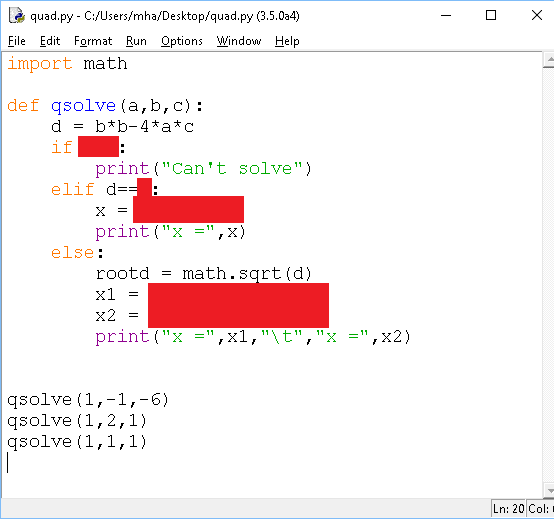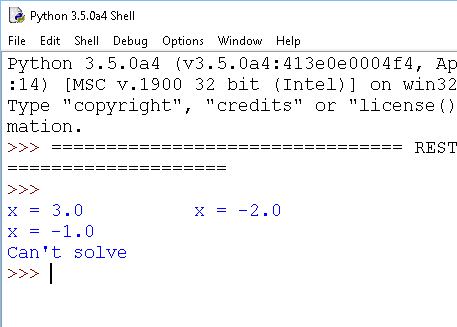# Python For GCSE Quadratic Equations

## Introduction

x2 - x - 6

In Maths, you might normally solve this by factorising.

(x+2)(x-3)=0

This would give you the following solutions,
x=-2,x=3

Look at the graph we get when we plot the function, y = x2 - x - 6,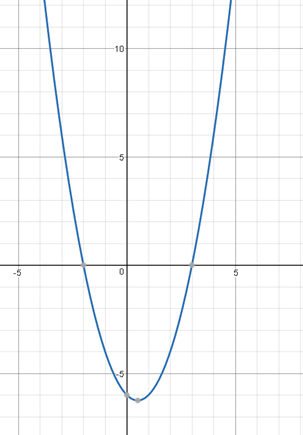The shape of the graph is called a parabola. If the coefficient of x2 is negative, the parabola would be inverted. This parabola intercepts the x axis (y=0) at two points, the two solutions to the equation.

x2 + 2x + 1

(x + 1)(x + 1)

You only get a single solution, x=-1

When we draw the graph, this time the curve touches the x axis at its lowest point (highest point if the parabola is inverted).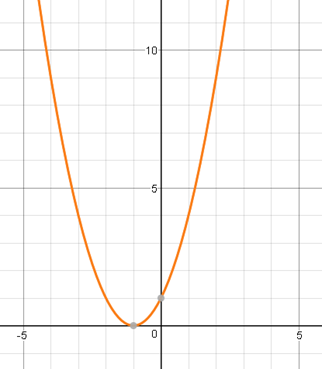When we write a program to solve quadratic equations, we will need to deal with the fact that some quadratic equations only have a single solution.

x2 + x + 1

This equation cannot be factorised. There is no way that the -1 and 1 can be added or subtracted to make a total of 1.

Looking at the graph, we can see that the parabola does not intercept the x axis. We can’t solve the equation.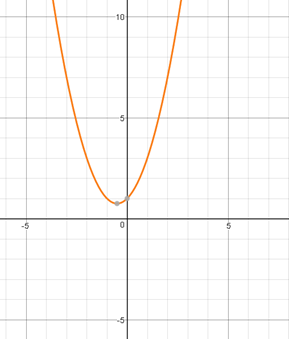Our program is going to have to be able to cope with this third type of equation too.

Given a quadratic equation expressed in the form,

ax2 + bx + c

The solutions can be directly calculated with the formula,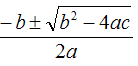The following shows how we use the formula for our three types of equation,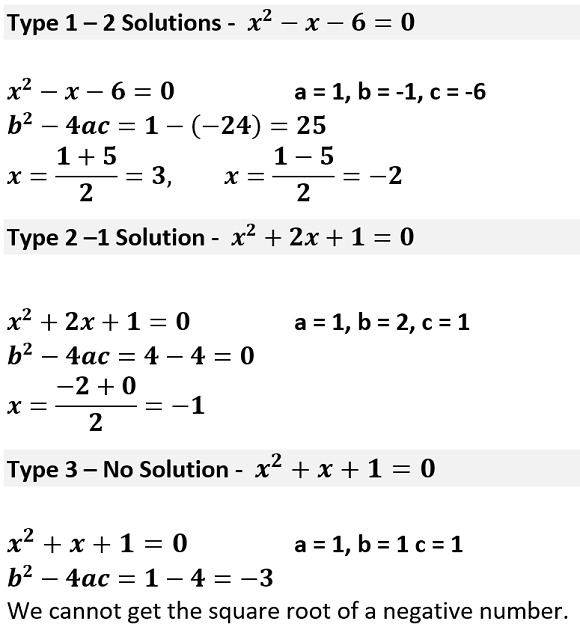### Programming

The part of the formula that matters most is b2 - 4ac. This is known as the discriminant of the equation.

Our program needs to do something like the following,

```d = b * b - 4 * a * c IF d < 0 THEN    OUTPUT "Cannot Solve" ELSE IF d == 0 THEN    x = -b / (2 * a)    OUTPUT "x = " + x ELSE    rootd = square root of d    x1 = (-b + rootd)/(2 * a)    x1 = (-b - rootd)/(2 * a)    OUTPUT "x = " + x1 + ", x = " + x2 END IF```

And here is an outline in Python with some strategic splodges,